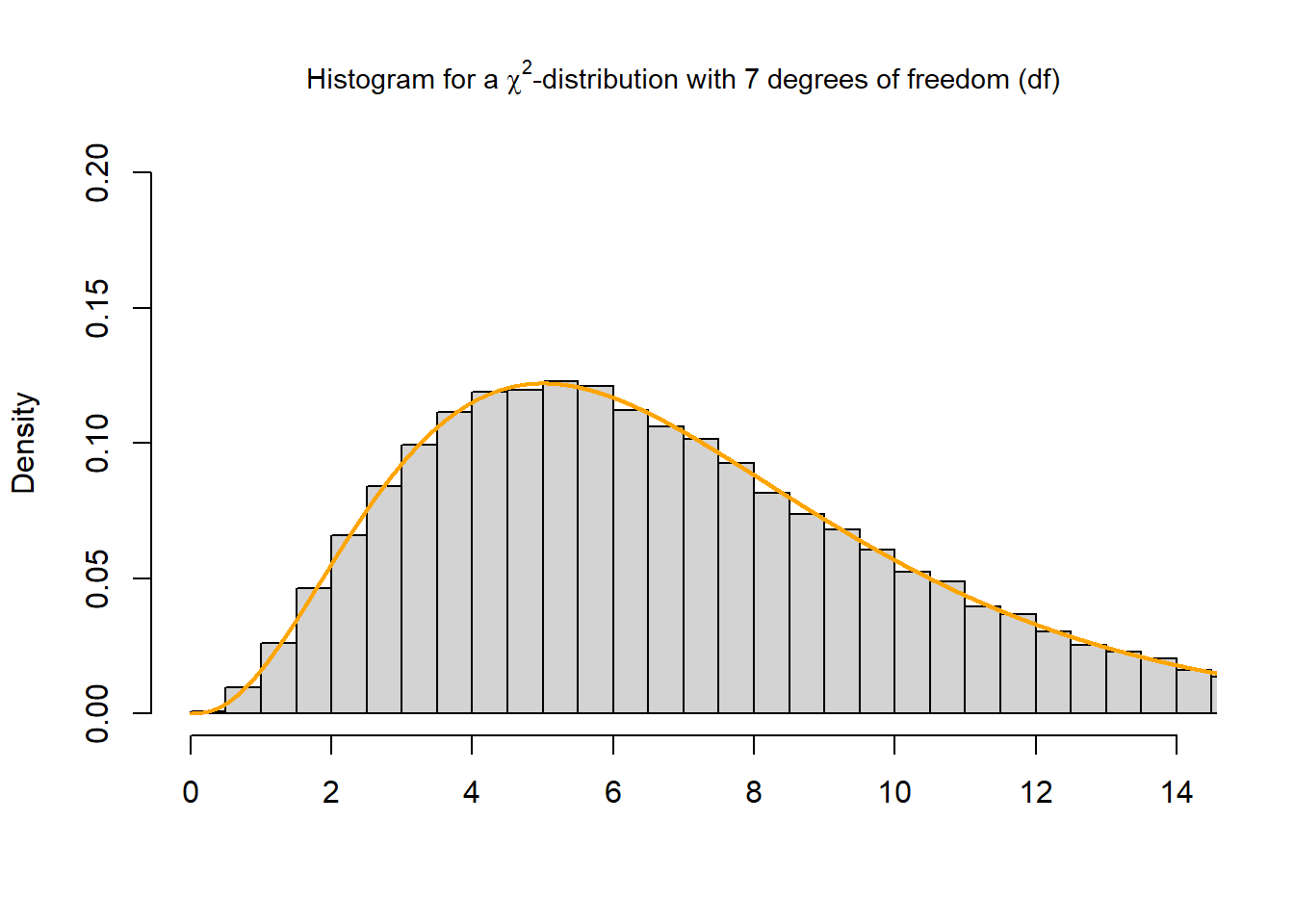The main functions using the $$\chi^2$$-distribution in R are dchisq(), pchisq(), qchisq() and rchisq(). The dchisq() function gives the density, the pchisq()function gives the distribution function, the qchisq() function gives the quantile function and the rchisq()function generates random deviates.

We use the dchisq() function to calculate the density of a $$\chi^2$$-curve with $$df=7$$ for the integer values 4 to 8:

dchisq(4:8, df = 7)
##  0.11518073 0.12204152 0.11676522 0.10411977 0.08817914

We use the pchisq() function to calculate the area under the curve for the interval $$[0,6]$$ and the interval $$[6, \infty)$$ of a $$\chi^2$$-curve with $$df=7$$:

# interval $[0,6] pchisq(6, df = 7, lower.tail = TRUE) ##  0.4602506 # interval$[6,inf]
pchisq(6, df = 7, lower.tail = FALSE)
##  0.5397494

Further, we check if the sum of the intervals $$[0,6]$$ and $$[6, \infty)$$ sums up to 1:

pchisq(6, df = 7, lower.tail = TRUE) + pchisq(6, df = 7, lower.tail = FALSE) == 1
##  TRUE

We use the qchisq() function to calculate the quantile for a given area (= probability) under the curve, that corresponds to $$q = 0.25, 0.5, 0.75$$ and $$0.999$$, for a $$\chi^2$$-curve with $$df=7$$. We set lower.tail = TRUE in order the get the area for the interval $$[0, q]$$.

qchisq(0.25, df = 7, lower.tail = TRUE)
##  4.254852
qchisq(0.5, df = 7, lower.tail = TRUE)
##  6.345811
qchisq(0.75, df = 7, lower.tail = TRUE)
##  9.037148
qchisq(0.999, df = 7, lower.tail = TRUE)
##  24.32189

Finally, we use the rchisq() function to generate 100,000 random values from the $$\chi^2$$-distribution with $$df=7$$. Thereafter, we plot a histogram and compare it to the probability density function of the $$\chi^2$$-distribution with $$df=7$$ (orange line):

x <- rchisq(100000, df = 7)
hist(x,
breaks = "Scott",
freq = FALSE,
xlim = c(0, 14),
ylim = c(0, 0.2),
xlab = "",
main = (TeX("Histogram for a $\\chi^2$-distribution with 7 degrees of freedom (df)")), cex.main = 0.9
)

curve(dchisq(x, df = 7), from = 0, to = 15, n = 5000, col = "orange", lwd = 2, add = T)